## Fast projection onto a Simplex (Python)

(work in progress)

Many convex optimization problems can be solved, fairly simply, if the projection onto the constraints can be quickly and simply calculated. By projection onto the constraints, we mean the solution to the following optimization problem:

(1)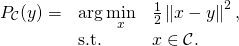As an example, the subgradient descent method can incorporate the projection operator to deal with constraints. Here we focus on projection on a simplex: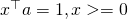. The objective function that we wish to solve is:

(2)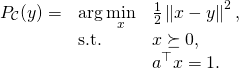As a test case, we can implement the projection in cvxpy:

import cvxpy as cvx
import numpy as np

def proj_simplex_cvxpy(a, y):
'''
Returns the point in the simplex a^Tx = 1, x&amp;amp;amp;amp;gt;=0 that is
closest to y (according to Euclidian distance)
'''
d = len(a)

# setup the objective and constraints and solve the problem
x = cvx.Variable(d)
obj = cvx.Minimize(cvx.sum_squares(x - y))
constr = [x &gt;= 0, a*x == 1]
prob = cvx.Problem(obj, constr)
prob.solve()

return np.array(x.value).squeeze()


We can write the dual as:Since the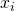decouples (for fixed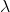) it can be solved as: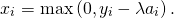The dual objective (which is to be maximized) is therefore:An example of the dual function is given below (source code is here):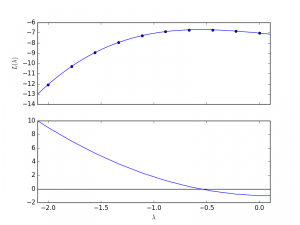Asis decreased from a large value, the values ofwill “switch on” from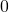. To determine where the variables will switch on we sort the values according to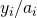.
Consider that we have an index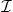that sorts according to. In other words: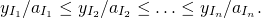We can refer to these as our indexedvalues,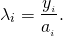Suppose that we have avalue. We then want to find an index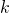, so that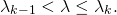We can then write the objective function as: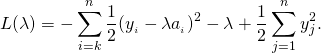The derivative of the above is therefore: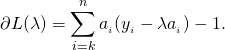By searching on the sorted list, we get an index, such that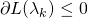, but.
The optimal lambda value can then be calculated as: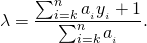The final algorithm is:

def proj(a, y):

l = y/a
idx = np.argsort(l)
d = len(l)

evalpL = lambda k: np.sum(a[idx[k:]]*(y[idx[k:]] - l[idx[k]]*a[idx[k:]]) ) -1

def bisectsearch():
idxL, idxH = 0, d-1
L = evalpL(idxL)
H = evalpL(idxH)

if L<0:
return idxL

while (idxH-idxL)>1:
iMid = int((idxL+idxH)/2)
M = evalpL(iMid)

if M>0:
idxL, L = iMid, M
else:
idxH, H = iMid, M

return idxH

k = bisectsearch()
lam = (np.sum(a[idx[k:]]*y[idx[k:]])-1)/np.sum(a[idx[k:]])

x = np.maximum(0, y-lam*a)

return x


## Other resources:

• MATLAB implementation of projection onto unit simplex (description here)
• Michelot, C. (1986). A finite algorithm for finding the projection of a point onto the canonical simplex of∝ n. Journal of Optimization Theory and Applications, 50(1), 195-200.

### Todo:

1. Implement the code in C/C++
2. Give a better test case
3. Simplify the explanation and implementation
4. Give a practical example (e.g. estimation problem with subgradient descent)